# Phase Resolved Spectroscopy## Overview

#### Synopsis:

Creating a phase-resolved spectrum for a periodic source allows the user to compare the spectrum from different portions of the cycle and look for changes that may occur as the source rotates or orbits.

This thread has been completely re-written. Previous versions of this thread used a different technique to filter the event file on PHASE that left the user to compute the correct exposure time. This new technique provides a way to filter the data using good time intervals (GTIs) so that the Datamodel can compute the exposure time automatically. This version of the thread also provides some guidance on how to determine the period.

#### Purpose:

To create a phase-resolved spectrum for a source.

Last Update: 7 2022 - Review for CIAO 4.14. Updated for Repro5 and CALDB 4.9.6.

## Get Started

`unix% download_chandra_obsid 2795 `Note

Users should not fold a spectrum on a period that is close to or smaller than the temporal resolution of the data. The resolution is the TIMDEL value in the header of the event file.

```unix% dmkeypar acisf02795_repro_evt2.fits TIMEDEL echo+
0.44104
```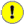Gratings Data

Users working with gratings data should pay extra attention to the dmcopy opt=all bug when working with TIME/GTI filters.

For the remainder of this thread it is assumed that the data have been reprocessed using chandra_repro and that all the files have been copied into the current working directory.

The Orbit Ephemeris file, orbitf121694700N001_eph1.fits, is included in the primary/ directory. Users with recently obtained datasets may only have access to the Level 0 ephemeris file (eph0.fits). This is the predicted ephemeris. The level 1 ephemeris (eph1.fits) is the definitive ephemeris which is generally available within about a month of the observation.

The data for this observation are shown in Figure 1. To simplify later commands, the event file is filtered with the region file and on energy

```unix% dmcopy "acisf02795_repro_evt2.fits[sky=region(ciao.reg),energy=500:7000]" RXJ0806.3+1527.evt
```

### Figure 1: Image of OBS_ID 2795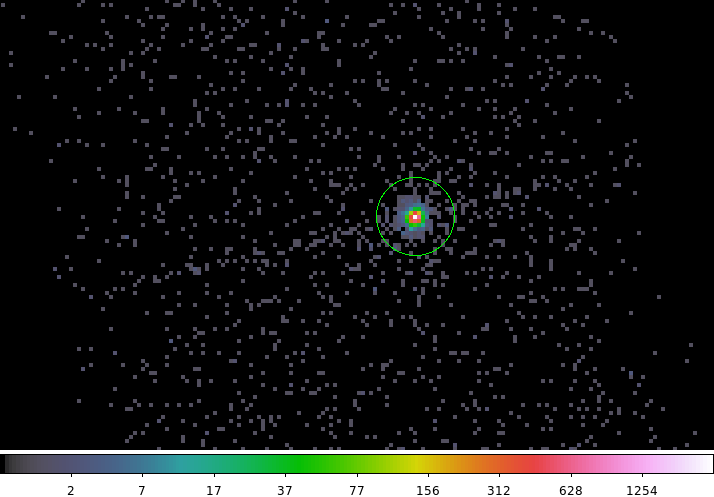### Figure 1: Image of OBS_ID 2795

An image of the level 2 event file with the source region shown. The region has been saved in CIAO format in physical coordinates and is shown here:

```unix% cat ciao.reg
circle(4144.93,4041.9479,10.0)
```

The observation is a single CCD, 128 row subarray. Given the location of the source it could dither off the subarray for part of the observation. This can impart a 707 or 1000 second periodicity onto the data. Any frequencies comparable to the dither frequency should be highly scrutinized. Users can check for the source region dithering off the active part of the detector using the dither_region tool. The Determining Exposed Region Area thread provides examples of using dither_region as does the Search for Variability in a Source thread.

Most ACIS observations are performed with dither period in pitch and yaw of 707.1 and 1000.0 seconds. For HRC the standard dither is performed with periods of 768.6 and 1087.0 seconds. The WebChaser Details page for an observation will contain the dither periods for any observation performed using non-standard dither parameters.

The filtered version of the event file is used in the remainder of this thread.

## Apply Barycenter Correction

The times in the data products need to be corrected to barycenter following the applying a barycenter correction thread. This is to account for the difference in photon arrival times as Chandra orbits the Earth and the Earth moves around the Sun. This barycenter correction should precede any phase calculation when trying to align phase between observations.

A summary of the steps in the thread are shown below.

### Correct the event file and GTIs

```unix% axbary RXJ0806.3+1527.evt orbitf121694700N001_eph1.fits RXJ0806.3+1527_bary.evt ra=121.5956933655199 dec=15.45862251788717
```

### Correct the aspect solution file

```unix% axbary pcadf121941470N004_asol1.fits orbitf121694700N001_eph1.fits RXJ0806.3+1527_bary.asol ra=121.5956933655199 dec=15.45862251788717
```

If the observation has multiple aspect solution files, this correction must be applied to each individually. The new ASOL file name is now added to the header of the event file so that later tools can automatically locate it.

```unix% dmhedit RXJ0806.3+1527_bary.evt file= op=add key=asolfile value=RXJ0806.3+1527_bary.asol
```

### Correct the Exposure Statistics file

For ACIS observations, there is one additional data product that must be barycenter corrected: the exposure statistics, stat1 file:

```unix% axbary acisf02795_000N003_stat1.fits orbitf121694700N001_eph1.fits.gz RXJ0806.3+1527_bary.expstats ra=121.5956933655199 dec=15.45862251788717
```

This file will be used to correctly align the good time intervals (GTIs) with ACIS exposure frame time boundaries.

## Determining the Period

Users who know the period of their source can skip directly to the Compute Phase section of this thread.

CIAO provides some tools to perform rudimentary timing analysis that can be used to determine the periodicity of a variable source. This section highlights those tools and provides some insight into how to interpret the outputs.

There are several papers in the literature about this source which all cite a period around 321 seconds:

which provide independent verification of the results presented here.

### Light Curves

dmextract can be used to extract a light curve binned at a fixed time resolution. Below is an example using a bin size of 20 seconds. The output lightcurve is shown in Figure 2. Remember that the event file used here has already been filtered spatially and by energy range.

```unix% dmextract "RXJ0806.3+1527_bary.evt[bin time=::20]" RXJ0806.3+1527.lc op=ltc1 clob+
```

Background has been ignored in this example due to the brightness of the source and the small region size.

### Figure 2: Light Curve for RXJ0806.3+1527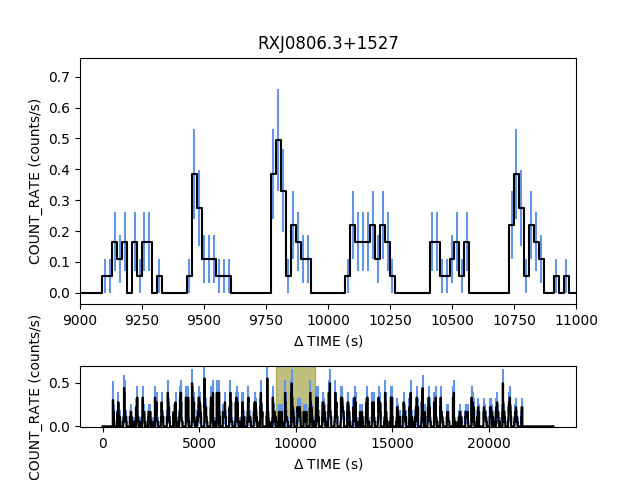### Figure 2: Light Curve for RXJ0806.3+1527

Light curve for RXJ0806.3+1527 binned at 20sec resolution. The top plot is a zoomed in view of the highlighted part of the full lightcurve shown in the bottom plot. The python commands to create the plot using matplotlib are shown below:

```from pycrates import read_file
import matplotlib.pylab as plt

x = tab.get_column("dt").values
y = tab.get_column("count_rate").values
ye = tab.get_column("count_rate_err").values

grid = plt.GridSpec(6,1,wspace=0.0, hspace=0.0)
plt.subplot(grid[0:4,0])

plt.errorbar( x, y, yerr=ye, color="black", marker="None", ecolor="cornflowerblue", drawstyle="steps-mid")
plt.xlim(9000,11000)
plt.ylabel("COUNT_RATE (counts/s)")
plt.xlabel(r"\$\Delta\$ TIME (s)")
plt.title("RXJ0806.3+1527")

plt.subplot(grid[5,0])
plt.errorbar( x, y, yerr=ye, color="black", marker="None", ecolor="cornflowerblue", drawstyle="steps-mid")
plt.fill([9000,11000,11000,9000],[-1,-1,1,1],color="olive", alpha=0.5)
plt.ylim(-0.01, 0.7)
plt.ylabel("COUNT_RATE (counts/s)")
plt.xlabel(r"\$\Delta\$ TIME (s)")
```

The choice of bin size can sometimes mask variability or accentuate artificial/instrumental frequencies. The next tool uses a Bayesian blocks approach to determine the optimal bin size.

### Gregory-Loredo Bayesian Blocks

The glvary tool creates a probability weighted, optimally binned lightcurve using the Gregory-Loredo Bayesian blocks algorithm.

```unix% glvary RXJ0806.3+1527_bary.evt outfile=glvary.prob lcfile=glvary.lc effile="" clob+
```

The data are shown in Figure 3.

### Figure 3: Gregory-Loredo Lightcurve for RXJ0806.3+1527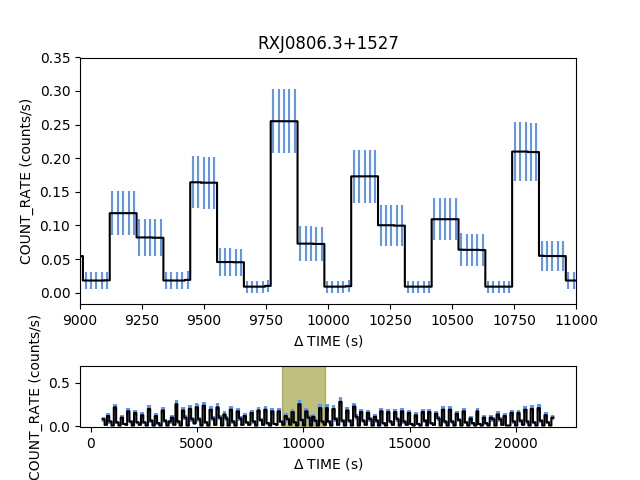### Figure 3: Gregory-Loredo Lightcurve for RXJ0806.3+1527

The Baysian Blocks, Gregory-Loredo lightcurve for RXJ0806.3+1527. The top plot is a zoomed in view of the highlighted part of the full lightcurve shown in the bottom plot. The python commands to create the plot are shown below:

```from pycrates import read_file
import matplotlib.pylab as plt

x = tab.get_column("time").values
x=x-121941081.557       # shift to match dmextract dt values
y = tab.get_column("count_rate").values
ye = tab.get_column("count_rate_err").values

grid = plt.GridSpec(6,1,wspace=0.0, hspace=0.0)
plt.subplot(grid[0:4,0])

plt.errorbar( x, y, yerr=ye, color="black", marker="None", ecolor="cornflowerblue", drawstyle="steps-mid")
plt.xlim(9000,11000)
plt.ylabel("COUNT_RATE (counts/s)")
plt.xlabel(r"\$\Delta\$ TIME (s)")
plt.title("RXJ0806.3+1527")

plt.subplot(grid[5,0])
plt.errorbar( x, y, yerr=ye, color="black", marker="None", ecolor="cornflowerblue", drawstyle="steps-mid")
plt.fill([9000,11000,11000,9000],[-1,-1,1,1],color="olive", alpha=0.5)
plt.ylim(-0.01, 0.7)
plt.ylabel("COUNT_RATE (counts/s)")
plt.xlabel(r"\$\Delta\$ TIME (s)")
```

The same range is highlighted as in Figure 2.

Figure 3 closely resembles Figure 2. The glvary curve is more smooth since it is computed by summing probability weighted lightcurves at different resolutions. Users will also note that the curve does not go to zero which is a byproduct of the GL algorithm.

The Search for Variability in a Source thread shows more examples of how to use the glvary tool.

Having established that the source is periodic, attention now turns to determining the frequency.

### Power Spectrum

The standard approach to determine the frequency for a periodic signal is to compute the power spectrum (amplitude of the Fourier transform). Users should in general be cautious when trying to interpret the power spectrum of a lightcurve for low count data; Poisson noise can easily mask faint signals. This dataset has a significant number of counts per bin so the power spectrum is meaningful.

The dmextract lightcurve should be used to create the power spectrum as the glvary lightcurve has been too heavily processed. Also, although not a concern here, dmextract can produce a background subtracted lightcurve while glvary cannot (at least not directly).

First, the mean count rate is subtracted from the lightcurve. This is to suppress the large, constant, spike at 0 frequency that can obscure low frequency components.

```unix% dmstat "RXJ0806.3+1527.lc[cols count_rate]"
COUNT_RATE[count/s]
min:	0 	      @:	1
max:	0.5513 	      @:	265
mean:	0.072791138986
sigma:	0.10489306672
sum:	85.037711491
good:	1169
null:	0

unix% set meanval = `pget dmstat out_mean`
unix% dmtcalc RXJ0806.3+1527.lc RXJ0806.3+1527_mean_offset.lc exp="count_rate=count_rate-\${meanval}" clob+
```

Then the power spectrum is computed using the apowerspectrum tool.

```unix% apowerspectrum infilereal="RXJ0806.3+1527_mean_offset.lc[cols time,count_rate]" infileimag=none outfile=RXJ0806.3+1527.power clob+
unix% dmlist RXJ0806.3+1527.power cols

--------------------------------------------------------------------------------
Columns for Table Block POWERSPECTRUM
--------------------------------------------------------------------------------

ColNo  Name                 Unit        Type             Range
1   FREQUENCY            (s)**-1      Real8          -Inf:+Inf
2   DATA                              Real4          -Inf:+Inf
```

Since the lightcurve is entirely real valued, use infileimag=none. The output file is simply Frequency versus Power (amplitude of the Fourier transform). The data are shown in Figure 4.

### Figure 4: Power Spectrum of Lightcurve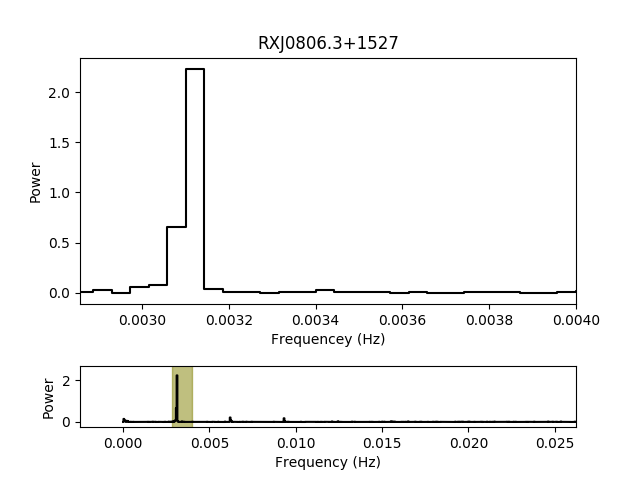### Figure 4: Power Spectrum of Lightcurve

The power spectrum for the mean-subtracted lightcurve (Figure 2). The full range of frequencies upto the Nyquist frequency are show in the inset, with the main plot zoomed in on the dominate spike.

The power peaks at a frequency between 0.00310 and 0.00314 Hz (period between 318.5 and 322.6 seconds).

The python commands to make the plot are shown below.

```from pycrates import read_file
import matplotlib.pylab as plt

x = tab.get_column("frequency").values
y = tab.get_column("data").values

grid = plt.GridSpec(6,1,wspace=0.0, hspace=0.0)
plt.subplot(grid[0:4,0])

plt.plot( x, y, color="black", marker="None", drawstyle="steps-mid")
plt.xlim(1.0/350, 1.0/250)
plt.ylabel("Power")
plt.xlabel("Frequencey (Hz)")
plt.title("RXJ0806.3+1527")

plt.subplot(grid[5,0])
plt.plot( x, y, color="black", marker="None", drawstyle="steps-mid")
plt.fill([1.0/350,1.0/350,1.0/250,1.0/250],[-1,10,10,-1],color="olive", alpha=0.5)
plt.ylim(-0.25, 2.7)
plt.xlim(right=0.026239)
plt.ylabel("Power")
plt.xlabel("Frequency (Hz)")
```

The power peaks at a frequency between 0.00310 and 0.00314 Hz (period between 318.5 and 322.6 seconds), with some higher frequency harmonics present. Those values agree well with the 321 second period reported in the literature.

To provide a better estimate of the frequency using the power spectrum approach would require using a smaller bin size with dmextract. However, the bin size needs to be significantly larger than the native time resolution (TIMEDEL=0.44 seconds) to avoid aliasing effects so this is about at the practical limit for this dataset.

Another common approach to locate the frequency of a periodic signal is to perform period folding (may also be called epoch folding). This is performed with the pfold tool.

### Period Fold

Another way to refine the period is to search for periods in some specified range. The basic algorithm is to iterate over a grid of expected periods, create a phased-binned lightcurve for each period, and the locate which period shows the strongest deviation from a uniform/flat signal. Folding lowers the statistical uncertainty in the phase bins as the number of counts increase.

There are various metrics that can be used to measure strongest, ie the L-statistic used in the SITAR package. pfold currently only outputs the standard deviation of the count rate, SIGMA_RATE, together with the average count rate MEAN_RATE.

To ensure that all the products are phase aligned (using the same time offset for PHASE=0), the TSTART value from the event file header is explicitly being used.

```unix% dmkeypar RXJ0806.3+1527_bary.evt tstart echo+
121941081.557
```

The power spectrum identified a periodic signal with a period of ~320 second, so a grid of times around that period will be searched. This example uses a very generous range of periods from 300 to 400 seconds with a step size of 0.01 seconds.

```
unix% pfold RXJ0806.3+1527_bary.evt RXJ0806.3+1527.fold periodgrid=300:400:0.01 tzero=121941081.557 clobber=yes
unix% dmlist RXJ0806.3+1527.fold cols
--------------------------------------------------------------------------------
Columns for Table Block RXJ0806.3+1527.fold
--------------------------------------------------------------------------------

ColNo  Name                 Unit        Type             Range
1   PERIOD               sec          Real8          -Inf:+Inf            Folded Period
2   PHASE_MID                     Real8(10)      -Inf:+Inf            PHASE GRID
3   COUNTS                        Int4(10)       -                    Counts in each phase bin
4   RATE             counts/sec   Real8(10)      -Inf:+Inf            Folded rates
5   ONTIME           sec          Real8(10)      -Inf:+Inf            Exposure time
6   DTF                           Real8(10)      -Inf:+Inf            Deadtime correction
7   MEAN_RATE            counts/sec   Real8          -Inf:+Inf            Average rate
8   SIGMA_RATE           counts/sec   Real8          -Inf:+Inf            Stdev of rate
```

The pfold output contains the phase binned lightcurve (PHASE_MID vs. RATE) for each of the input periods. Additionally the MEAN_RATE (average count rate) and SIGMA_RATE (standard deviation of count rate values) is reported; these values shown in Figure 5.

### Figure 5: Period Fold Results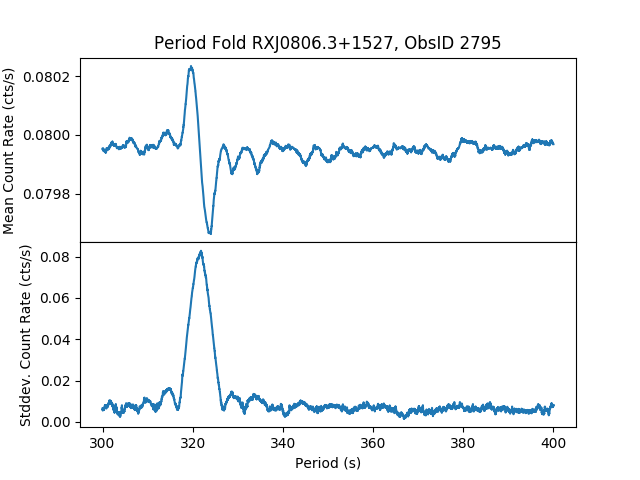### Figure 5: Period Fold Results

The pfold output for the MEAN_RATE (top) and SIGMA_RATE (bottom) verses period. The characteristic sinc (sin(x)/x) shape in the SIGMA_RATE curve is strongly indicative of periodic signal with frequency at the maximum (that is the maximum deviation from a flat/constant signal). The peak occurs at a period of 321.85 seconds. The inset plot shows the phase binned lightcurve at 321.85 seconds.

The python commands to make this plot is shown here:

```from pycrates import read_file
import matplotlib.pyplot as plt

period=tab.get_column("period").values
mean_rate=tab.get_column("mean_rate").values
sigma_rate=tab.get_column("sigma_rate").values

plt.subplot(2,1,1)
plt.plot(period,mean_rate, marker="None")
plt.ylabel("Mean Count Rate (cts/s)")
plt.title("Period Fold RXJ0806.3+1527, ObsID 2795")

plt.subplot(2,1,2)
plt.plot(period,sigma_rate, marker="None")
plt.ylabel("Stddev. Count Rate (cts/s)")
plt.xlabel("Period (s)")
```

The peak in the SIGMA_RATE column occurs at a period of 321.85 seconds. The phase binned lightcurve for that period is shown in the Figure 6 inset.

### Figure 6: Period Fold Lightcurve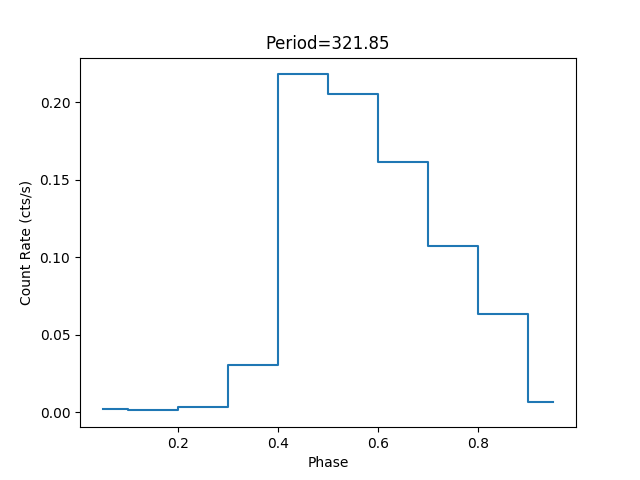### Figure 6: Period Fold Lightcurve

Folded lightcurve at period=321.85.

```from pycrates import read_file
import matplotlib.pyplot as plt

x=tab.get_column("phase_mid").values.flatten()
y=tab.get_column("rate").values.flatten()

plt.plot(x,y,marker="None", drawstyle="steps-mid")
plt.title("Period=321.85")
plt.xlabel("Phase")
plt.ylabel("Count Rate (cts/s)")
```

Analysis of the pfold output shows that a period of 321.85 seconds should be used for to compute the PHASE.dmtype2split

The pfold array columns can be converted into normal scalar arrays using the dmtype2split tool. While this tool is normally used to convert gratings type II PHA files into standard type I files, it can be used more generically to convert any file with array columns into scalar columns.

```unix% dmtype2split RXJ0806.3+1527.fold"[period=321.85]" 321.85.fits
unix% dmlist 321.85.fits cols

--------------------------------------------------------------------------------
Columns for Table Block 321.85.fits
--------------------------------------------------------------------------------

ColNo  Name                 Unit        Type             Range
1   PHASE_MID                         Real8          -Inf:+Inf
2   COUNTS                            Int4           -
3   RATE                 counts/sec   Real8          -Inf:+Inf
4   ONTIME               sec          Real8          -Inf:+Inf
5   DTF                               Real8          -Inf:+Inf
```

This file format may be easier for other applications to use.

## Compute Phase

In the previous section several types of analysis were performed to come to the conclusion that RXJ0806.3+1527 has a period of 321.85 seconds. This number will now be used to create a set of Good Time Intervals (GTIs) and to extract a spectra from the brightest part of the cycle.

### Period fold expression

This thread will be using dmtcalc to compute the phase (fraction of the period) of times in various data products. dmtcalc can compute arbitrary expressions via its rich expression syntax.

The expression to compute the phase is shown below and saved into a file named dmtcalc.expr

```unix% cat dmtcalc.expr
.period=321.85
.tzero=121941081.5572
.freq=(1.0/.period)
GPHASE=((time-(.tzero))*.freq)
PHASE=(GPHASE-((long)GPHASE))
```dmtcalc dot syntax

The leading dot (period) for the columns .period, .tzero, and .freq designate them as temporary columns which are not written to the output file.

If the frequency was known, instead of the period, then the .freq column can be set directly.

Folding the observation entails associating all the times that happen at a given point in the phase cycle with one another, with the phase traditionally being defined as a fraction of the orbital or rotational cycle < 1. To do this, the integer portion of the GPHASE must be subtracted off so that all values are greater than or equal to 0 and less than 1 (e.g. phases of 0.5, 1.5, and 2.5 all fall at identical points in the cycle):TSTART values

```GPHASE=((time-TSTART)*.freq)
```

This is a problem because the TSTART value is different in different data products. Be sure to use the same tzero value with all the files.

This dmtcalc.expr file can now be used as input to dmtcalc. First, the phase for the times in the event file are computed.

```unix% dmtcalc RXJ0806.3+1527_bary.evt RXJ0806.3+1527_phase.evt exp=@dmtcalc.expr clob+
unix% dmlist RXJ0806.3+1527_phase.evt cols
...
17   GPHASE                            Real8          -Inf:+Inf            User defined column
18   PHASE                             Real8          -Inf:+Inf            User defined column
...
```Frequency shifts

The expression for phase can be modified to include a frequency shift, fdot, by replacing the GPHASE expression with a more general form:

```GPHASE=(time-(.tzero))*(.freq +(.fdot * (time-(.tzero))))"
```

and then dmextract is used to compute the phase binned lightcurve

```unix% dmextract "RXJ0806.3+1527_phase.evt[bin phase=0.0:1.0:0.1]" phase.lc op=generic clob+
```

This example bins the events into 10 phase bins (going from 0 to 1.0 in 0.1 (10%) period bins). Note that the data are not being binned on time, they are being binned on phase, so the output format must be set as op=generic, not op=ltc1. The phase binned lightcurve is shown in Figure 7.

### Figure 7: dmextract: 321.85 seconds period folded lightcurve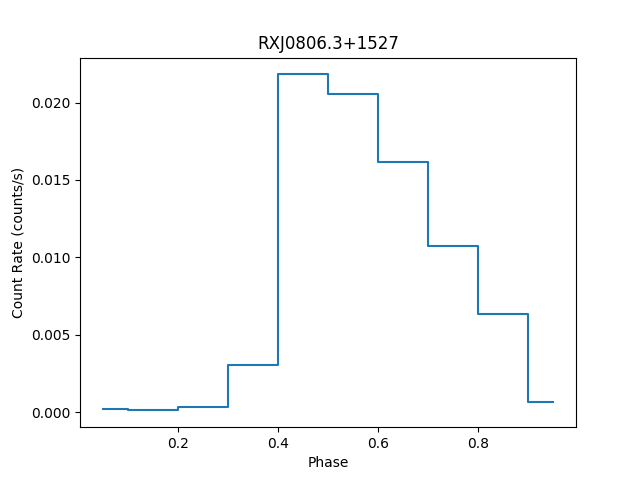### Figure 7: dmextract: 321.85 seconds period folded lightcurve

The phase binned lightcurve created with dmextract from the event files. Note the similarity to Figure 6. The shape is the same however the normalization is different by an order of magnitude.

```from pycrates import read_file
import matplotlib.pyplot as plt

x = tab.get_column("phase").values
y = tab.get_column("count_rate").values

plt.plot(x,y,drawstyle="steps-mid",marker="None")
plt.xlabel("Phase")
plt.ylabel("Count Rate (counts/s)")
plt.title("RXJ0806.3+1527")
```

While the shape of the curve is the same as the inset in Figure 5, we see that the COUNT_RATE is an order of magnitude lower. This is because the exposure time dmextract uses is the full length of the observation, instead of the per-phase-bin time. dmextract does not know that binning on PHASE implies a binning on TIME, nor does it know how to convert between the two.

For things to work out correctly, any expression involving phase needs to be expressed differently; it must be expressed as a function of time. This will be done by creating Good Time Interval (GTIs).

### Create Good Time Intervals (GTIs)

The correct way to filter/bin on phase is to convert PHASE into TIME. This will be accomplished using dmgti. The input to dmgti is a file with a TIME column. For phase binning the time resolution should be smaller than the time resolution of the event file. The aspect solution has a time resolution of 0.256 seconds which meets this need.

First, the PHASE for the times in the barycenter corrected aspect file are computed using dmtcalc as was done earlier with the event file.

```unix% dmtcalc RXJ0806.3+1527_bary.asol RXJ0806.3+1527_phase.asol exp=@dmtcalc.expr clob+
```

The next step is to determine the good times. Suppose for this example the goal was to anlyze the data when the source is brightest. Looking at Figure 5 this corresponds to a PHASE between 0.4 to 0.9. These values are then used with dmgti to determine what times (good times) have 0.4 <= PHASE < 0.9

```unix% punlearn dmgti
unix% pset dmgti infile=RXJ0806.3+1527_phase.asol
unix% pset dmgti outfile=high.gti
unix% pset dmgti userlimit="((0.4<=phase)&&(phase<0.9))"
unix% pset dmgti clobber=yes
unix% dmgti
Input MTL file (RXJ0806.3+1527_phase.asol):
Output GTI file (high.gti):
User defined limit string (((0.4<=phase)&&(phase<0.9))):
```

dmgti uses the same mathematical expression syntax as dmtcalc.

Examining the output there are 67 time intervals where the PHASE is within the specified range.

```unix% dmlist high.gti blocks

--------------------------------------------------------------------------------
Dataset: high.gti
--------------------------------------------------------------------------------

Block Name                          Type         Dimensions
--------------------------------------------------------------------------------
Block    1: PRIMARY                        Null
Block    2: FILTER                         Table         1 cols x 0        rows
Block    3: GTI                            Table         2 cols x 67       rows
```

The times in the high.gti file now correspond to times when the phase is between 0.4 and 0.9.

### Align GTIs on ACIS exposure frame time boundaries

The next step is to align those GTI time boundaries (start and stop times) to match with the start and stop times of the actual ACIS exposure times. (This step is not necessary when working with HRC data.) This is a small correction to each GTI, just a fraction of the frame time; however, with many GTIs the cumulative adjustment can become significant.

The gti_align script is used to adjust the arbitrary phase times. The script uses the exposure statistics file, stat1.fits, which is why the barycenter time corrected were also applied to it.

```unix% pset gti_align times=high.gti
unix% pset gti_align statfile=RXJ0806.3+1527_bary.expstats
unix% pset gti_align outfile=high_align.gti
unix% pset gti_align evtfile=RXJ0806.3+1527_bary.evt
unix% gti_align mode=h clobber=yes
```

Figure 8 shows a comparison of the GTI START and STOP times after the aligmnent. Since the frame time is approximately 0.44 seconds, the amount each GTI is shifted in either side is no more than 0.44 seconds.

### Figure 8: GTI Alignment Corrections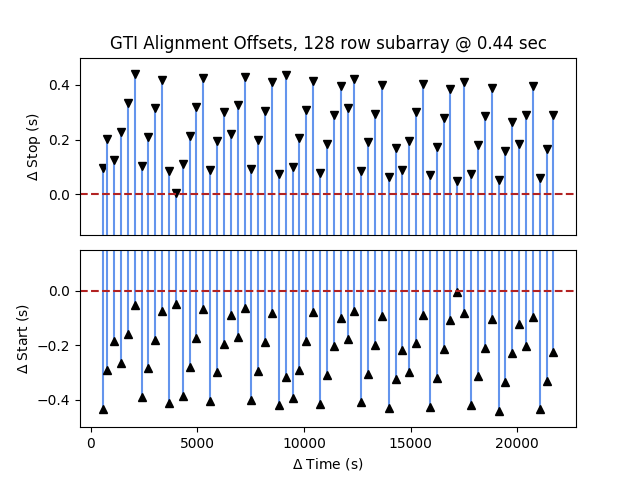### Figure 8: GTI Alignment Corrections

GTI alignment corrections. (Top) shows the correction to the STOP times while (Bottom) shows the correction to the START times. Either side is shifted by upto 0.44 (TIMEDEL) seconds. The following bit of Python can be used to plot the results

```from pycrates import read_file
import matplotlib.pylab as plt

orig_lo = orig.get_column("start").values-121941081.557
orig_hi = orig.get_column("stop").values-121941081.557
lo = fix.get_column("start").values-121941081.557
hi = fix.get_column("stop").values-121941081.557

plt.subplot(2,1,1)

for l,h,ol,oh in zip( lo, hi, orig_lo, orig_hi ):
plt.plot([ol, ol], [l-oh, h-oh], marker="v", color="cornflowerblue",mfc="black",mec="black" )

plt.ylabel(r"\$\Delta\$ Stop (s)")
plt.title("GTI Alignment Offsets, 128 row subarray @ 0.44 sec")
plt.ylim(-0.15, 0.5)
plt.axhline(0, color="firebrick", linestyle='--' )
plt.gca().set_xticks([]) # hide x-ticks here

plt.subplot(2,1,2)

for l,h,ol,oh in zip( lo, hi, orig_lo, orig_hi ):
plt.plot( [ol, ol], [l-ol, h-ol], marker="^", color="cornflowerblue",mfc="black",mec="black" )

plt.ylabel(r"\$\Delta\$ Start (s)")
plt.xlabel(r"\$\Delta\$ Time (s)")
plt.ylim( -0.5, 0.15)
plt.axhline(0, color="firebrick", linestyle="--")
```

The difference in exposure time can be seen when applying two different GTIs

```unix% dmkeypar "RXJ0806.3+1527_bary.evt[@high.gti]" ontime echo+
10601.9740087092
unix% dmkeypar "RXJ0806.3+1527_bary.evt[@high_align.gti]" ontime echo+
10633.0197870433
```

A thirty second difference in ONTIME is probably not significant for this observation. The effect, however, is cumulative. For other periodic sources with shorter periods, there will be more GTIs and the correction can become a significant fraction of the total exposure time.

### Display GTIs

The GTIs can be inspected before extracting the spectra by plotting them on top of the lightcurve. Figure 9 shows the lightcurve in Figure 2; the good times are the times shaded intervals.

### Figure 9: GTIs shown on lightcurve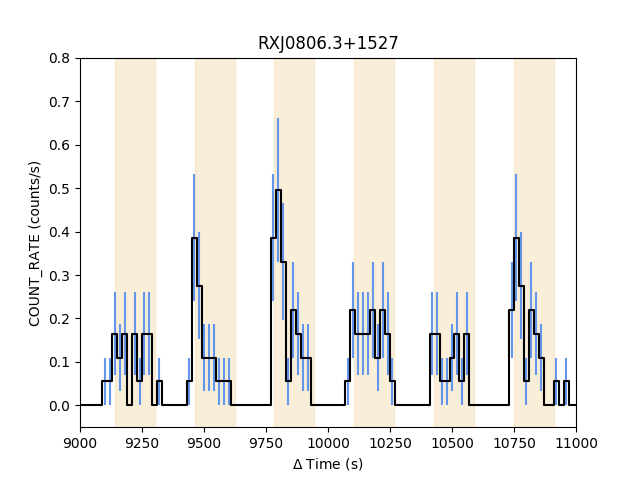### Figure 9: GTIs shown on lightcurve

The exposure frame time aligned GTIs shows on the zoomed in part of the lightcurve from Figure 2. The good times are the shaded time intervals.

The python commands to create this plot are shown here:

```from pycrates import read_file
import matplotlib.pylab as plt

xx = tab.get_column("dt").values
yy = tab.get_column("count_rate").values
ye = tab.get_column("count_rate_err").values
plt.errorbar( xx, yy, yerr=ye, color="black", marker="None", ecolor="cornflowerblue", drawstyle="steps-mid")

lo = gti.get_column("start").values-121941081.557
hi = gti.get_column("stop").values-121941081.557
for l,h in zip(lo,hi):
plt.fill( [l,l,h,h], [-1,1,1,-1], color="wheat", alpha=0.5)

plt.xlim(9000,11000)
plt.ylim(-0.05, 0.8)
plt.ylabel("COUNT_RATE (counts/s)")
plt.xlabel(r"\$\Delta\$ Time (s)")
plt.title("RXJ0806.3+1527")
```

## Extract Phase Resolved Spectrum

First, the event file is filtered with the GTI file. This is necessary to correctly set the EXPOSURE (and releated) keywords.

```unix% dmcopy RXJ0806.3+1527_bary.evt"[@high_align.gti]" RXJ0806.3+1527_high.evt clob+
```Gratings Data: tgextract/tgextract2

Users working with gratings data should follow the work around in the dmcopy opt=all bug when working with TIME/GTI filters.

Then specextract is used to extract the spectrum.

```unix% specextract "RXJ0806.3+1527_high.evt[sky=region(ciao.reg)]" RXJ0806.3+1527_high clob+

Running: specextract
Version:    date
Using event file RXJ0806.3+1527_high.evt[sky=region(ciao.reg)]

Aspect solution file RXJ0806.3+1527_bary.asol found.

Extracting src spectra

Creating src ARF

Creating src RMF

Using mkacisrmf...

Grouping src spectrum

Updating header of RXJ0806.3+1527_high.pi with RESPFILE and ANCRFILE keywords.

Updating header of RXJ0806.3+1527_high_grp.pi with RESPFILE and ANCRFILE keywords.
```

Even though the data have already been spatially filtered, specextract checks the infile for a spatial filter. Otherwise, applying the same filter twice has no effect.

As before with the lightcurve, background has been ignored when extracting the spectrum.

The spectrum can then be loaded into sherpa for modeling and fitting. The phase resolved spectrum is shown in Figure 10.

### Figure 10: Spectrum for Phase 0.4:0.9 with 321.85 second period.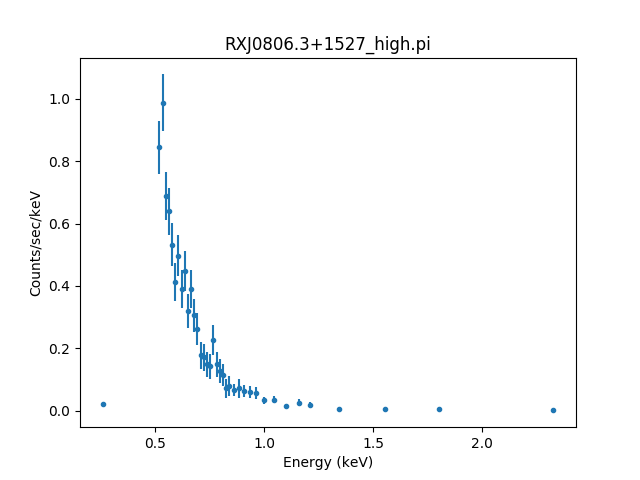### Figure 10: Spectrum for Phase 0.4:0.9 with 321.85 second period.

The spectrum for RXJ0806.3+1527 during the phase=0.4:0.9 interval of its 321.85 second periodicity. The data have been grouped to a minimum of 10 counts per channel.

The sherpa commands to load and display the data shown here:

```load_data("RXJ0806.3+1527_high.pi")
group_counts(10)
notice(0.3, 2.0 )
plot_data()
```

Users can now proceed directly to model and fit the data.

Since the PHASE was selected via GTIs, the EXPOSURE time has automatically been recomputed. The spectrum can now be modeled and fit.

## Summary

This thread analyzed the variable, periodic source RXJ0806.3+1527. The data products for the observation were barycenter corrected (axbary). Several timing tools were used to create lightcurves (dmextract and glvary) , search for variability (apowerspectrum), and determine the period of the variation (pfold). The period of 321.85 seconds agrees well with what is reported in the literature for this source in this observation. The data products were then phase folded using dmtcalc and a set of Good Time Intervals (GTIs) were created for the brightest part of the variation using dmgti. These GTIs were aligned to ACIS exposure frame time boundaries using the gti_align script. Finally these GTIs were used to filter the event file and specextract was used to extract the spectrum.